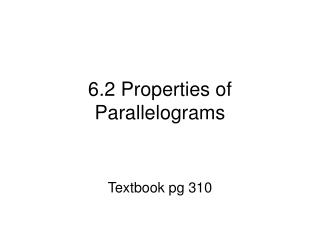DownloadDownload Presentation6.2 Properties of Parallelograms

# 6.2 Properties of Parallelograms

Download Presentation## 6.2 Properties of Parallelograms

- - - - - - - - - - - - - - - - - - - - - - - - - - - E N D - - - - - - - - - - - - - - - - - - - - - - - - - - -
##### Presentation Transcript

1. 6.2 Properties of Parallelograms Textbook pg 310

2. Definition: A Parallelogram is a quadrilateral where BOTH pairs of opposite sides are parallel • In PQRS, PQ SR and QR PS P Q R S

3. Theorem: If a quadrilateral is a parallelogram, then its opposite sides are congruent • In PQRS, PQ = SR and QR = PS P Q R S

4. Theorem: If a quadrilateral is a parallelogram, then its opposite angles are congruent • In PQRS, <P = <R and <Q = <S P Q R S

5. Theorem: If a quadrilateral is a parallelogram, then its consecutive angles are supplementary • In PQRS, xo + yo = 180o 180o P Q xo yo 180o 180o yo xo R S 180o

6. Theorem: If a quadrilateral is a parallelogram, then diagonals bisect each other • In PQRS, QM = MS and PM = MR 180o P Q 180o M 180o R S 180o

7. Practice • Assignment # • Textbook page 313 • Problems 1-12 all, 13-33 odd, 44 – 53 all